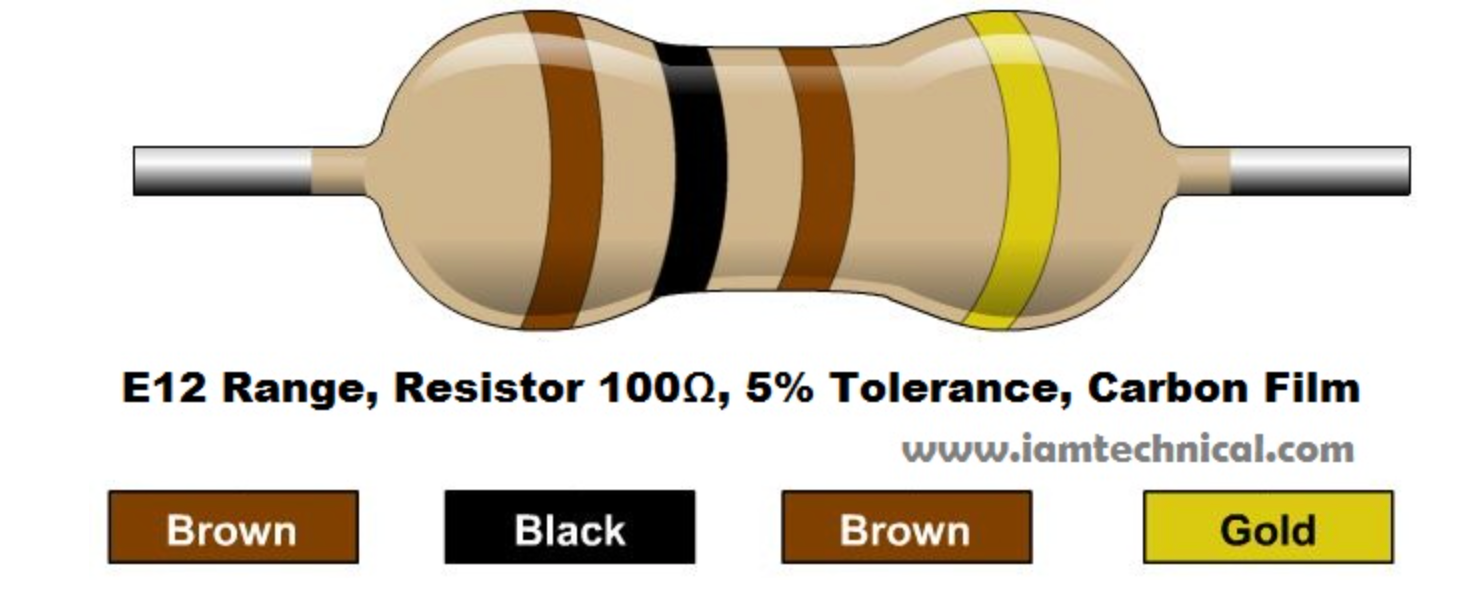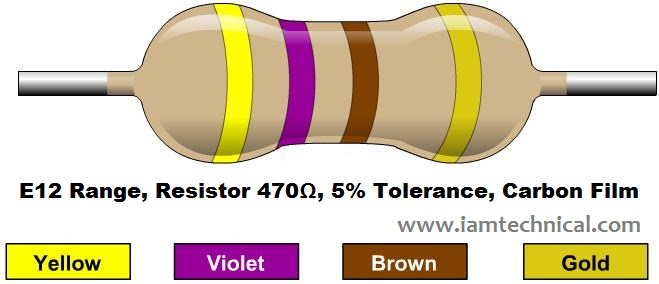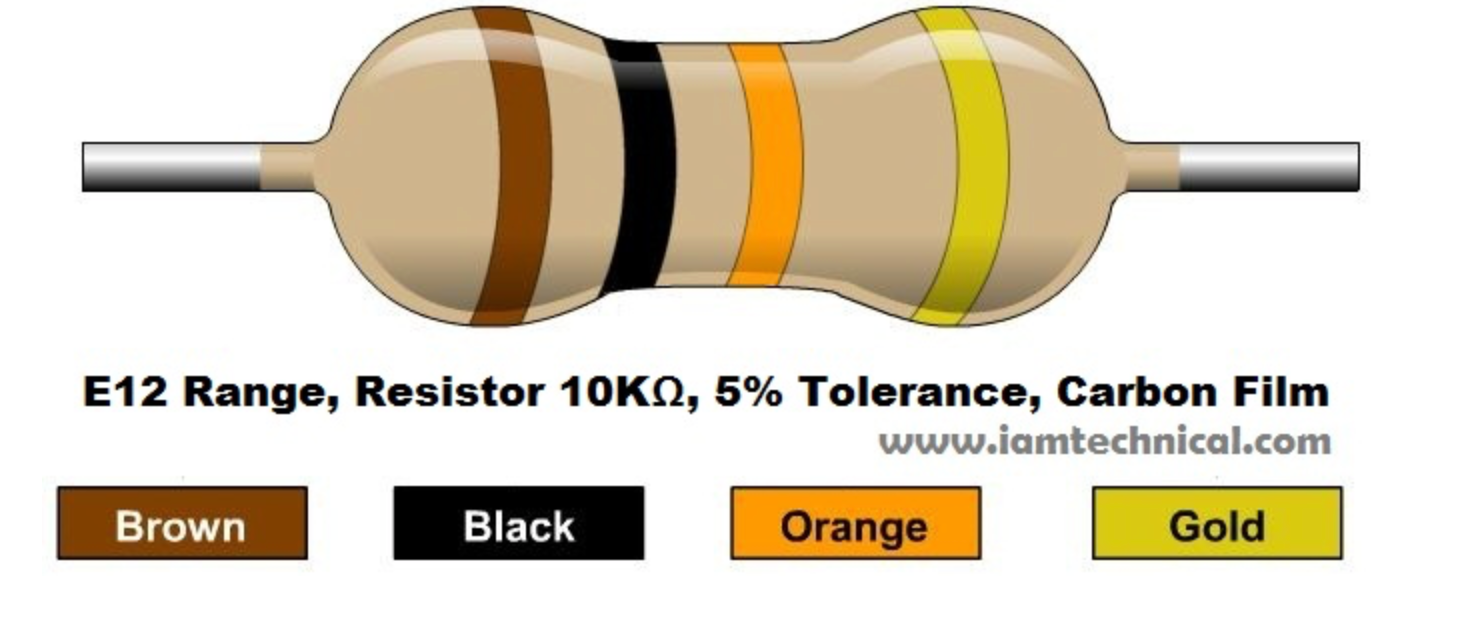# 9.1. Description

Resistors are electronic components that have specific, never-changing electrical resistance. They are resisting the flow of electrical energy in a circuit, changing the voltage and current as a result. Resistors are used to reduce current flow, adjust signal levels, to divide voltages, bias active elements, and terminate transmission lines, among other uses.
Resistors are passive components. It means they only consume power bat can’t generate it.

Its value is measured in ohms (Ω) - official SI unit of electrical resistance. Since resistors are specified and manufactured over a very large range of values, the derived units of milliohm (1 mΩ = 10−3Ω), kilohm (1 kΩ = 103Ω), and megohm (1 MΩ = 106Ω) are also in common usage.

The colored stripes on the sides of resistors indicate their value.

# 9.2. Ohm's law

The relationship between current, voltage and resistance is well known as Ohm's low. When you change one of these in a circuit it affects the others.

The behavior of an ideal resistor is dictated by the following relationship:

V = I x R

Where:
V = Voltage in volts
I = Current in amps
R = Resistance in ohms

Ohm's law states that the voltage (V) across a resistor is proportional to the current (I), where the constant of proportionality is the resistance (R).
For example, if a 300 ohm resistor is attached across the terminals of a 12 volt battery, then a current of 12 / 300 = 0.04 amperes flows through that resistor.

# 9.3. How to read resistor color code?

Most resistors are using a color-coding system. It means resistor values are marked out using bands, and each color corresponds to a number.
Each resistor has 4 or 5 bands. The first two bands (first three in case of 5 bands) indicate the two most-significant digits of the resistor’s value while the third one (the fourth one in case of 5 bands) is a weight value, which multiplies the two significant digits by a power of ten. The last band indicates the tolerance of the resistor. It explains how much more or less the actual resistance of the resistor can be compared to what its nominal value is.

See below 4 - band resistor color code identification:

### 📘Note

To build your air device you will need one 100Ω (RGB LED), one 470Ω (LED ring) and one 10kΩ (DHT22) resistor.

100Ω resistor looks like:470Ω resistor looks like:10kΩ resistor looks like: